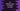# 4 ways in C# to reverse an array## Different ways in C# to reverse an Array:

In this post, we will learn different ways to reverse an Array in C#. We can iterate through the array in reverse and build a new array or we can use predefined methods. I will show you three different ways to reverse an array in this post.

### Method 1: By iterating through the array content in reverse:

In this method, we will iterate through the content of the array in reverse and build another array with its content.

``````using System;

namespace Program
{
class Program
{
static void Main(string[] args)
{
int[] GivenArray = { 1, 2, 3, 4, 5 };

int[] ReverseArray = new int[GivenArray.Length];

for (int i = GivenArray.Length - 1; i >= 0; i--)
{
ReverseArray[GivenArray.Length - i - 1] = GivenArray[i];
}

Console.WriteLine("Original Array: " + String.Join(" ", GivenArray));
Console.WriteLine("New Array: " + String.Join(" ", ReverseArray));
}
}
}``````

Here,

• GivenArray is the original array of integers.
• ReverseArray is another array. It is initialised as the equal length of the given array.
• The for loop is iterating through the GivenArray in reverse order. It inserts the numbers to the ReverseArray.
• The last two lines are printing the original and reverse arrays.

If you run this program, it will print the below output:

``````Original Array: 1 2 3 4 5
New Array: 5 4 3 2 1``````

### Method 2: By using a separate method:

The above program works only for integer arrays. We can also modify it with generics to work for any type of array. Let’s create a separate method to work with any type of array:

``````using System;

namespace Program
{
class Program
{
static T[] Reverse<T>(T[] Arr)
{
T[] ReverseArray = new T[Arr.Length];

for (int i = Arr.Length - 1; i >= 0; i--)
{
ReverseArray[Arr.Length - i - 1] = Arr[i];
}
return ReverseArray;
}
static void Main(string[] args)
{
int[] GivenArray = { 1, 2, 3, 4, 5 };
int[] ReverseArray = Reverse(GivenArray);

Console.WriteLine("Original Array: " + String.Join(" ", GivenArray));
Console.WriteLine("New Array: " + String.Join(" ", ReverseArray));
}
}
}``````

We are using generics in the Reverse method and it works with any type of array. We can pass an integer array, string array or any other type of array.

It will give the same output.

``````Original Array: 1 2 3 4 5
New Array: 5 4 3 2 1``````

### Method 3: By using Enumerable.Reverse():

Enumerable.Reverse() method can be used to create a reverse array. It doesn’t modify the original array. The following program reverse the content of the original array and creates a new array.

``````using System;
using System.Linq;

namespace Program
{
class Program
{
static void Main(string[] args)
{
int[] GivenArray = { 1, 2, 3, 4, 5 };
int[] ReverseArray = Enumerable.Reverse(GivenArray).ToArray();

Console.WriteLine("Original Array: " + String.Join(" ", GivenArray));
Console.WriteLine("New Array: " + String.Join(" ", ReverseArray));
}
}
}``````

It will give the same output.

### Method 4: By using Array.Reverse():

The Array.Reverse() is a method to do in-place reverse of the content of an array. It will overwrite the the existing content of the array without creating a new array. This is an inbuilt method and hence we can call it on any array variable.

``````using System;
using System.Linq;

namespace Program
{
class Program
{
static void Main(string[] args)
{
int[] GivenArray = { 1, 2, 3, 4, 5 };
GivenArray.Reverse();

Console.WriteLine("Original Array: " + String.Join(" ", GivenArray));
Console.WriteLine("New Array: " + String.Join(" ", GivenArray));
}
}
}``````

It will give the below result:

``````Original Array: 1 2 3 4 5
New Array: 1 2 3 4 5``````